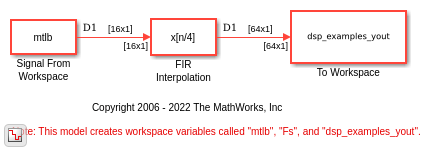# FIR Interpolation Using Single-Rate Processing

In this example, the FIR Interpolation block interpolates the signal by increasing the frame size, while keeping the same sample rate.

Open the model. The input to the FIR Interpolation block is a single-channel input with a frame size of 16. In the block dialog box, set the Interpolation factor parameter to 4 and the Rate options parameter to `Enforce single-rate processing`.

Run the model. The block operates in the single-rate processing mode. Therefore, the output frame size of the block is L times the input frame size of the block, where L is the interpolation factor. With an interpolation factor of 4, the output has a frame size of 64. As shown in the model, the input and the output of the FIR Interpolation block have the same sample rate but have different frame sizes.